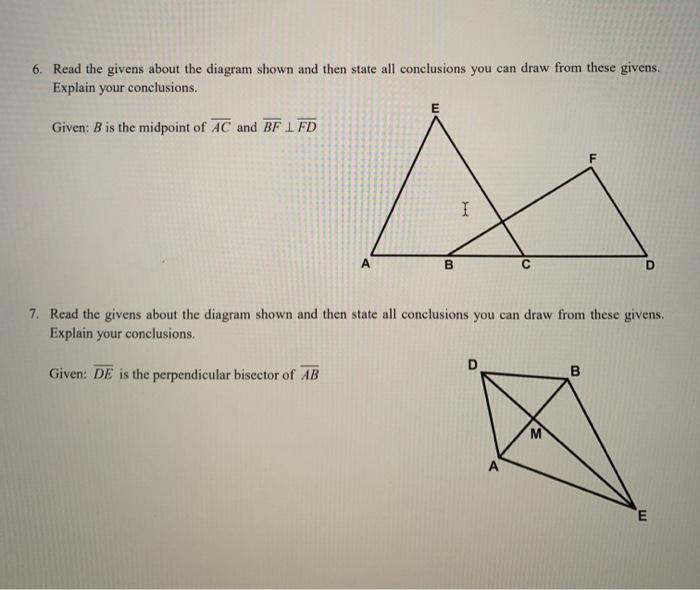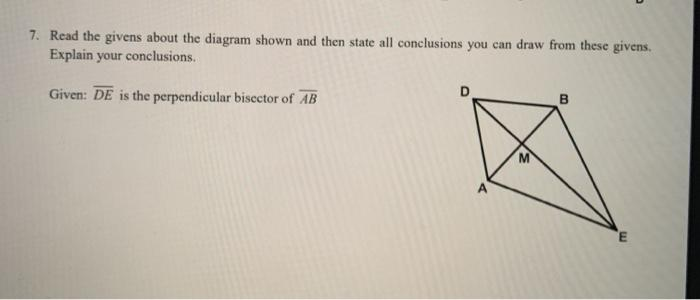# Question Solved1 AnswerONLY NUMBER 7 6. Read the givens about the diagram shown and then state all conclusions you can draw from these givens. Explain your conclusions. E Given: B is the midpoint of AC and BF 1 FD F I B D 7. Read the givens about the diagram shown and then state all conclusions you can draw from these givens Explain your conclusions. Given: DE is the perpendicular bisector of AB B M E 7. Read the givens about the diagram shown and then state all conclusions you can draw from these givens. Explain your conclusions. Given: DE is the perpendicular bisector of AB B M EONLY NUMBER 7Transcribed Image Text: 6. Read the givens about the diagram shown and then state all conclusions you can draw from these givens. Explain your conclusions. E Given: B is the midpoint of AC and BF 1 FD F I B D 7. Read the givens about the diagram shown and then state all conclusions you can draw from these givens Explain your conclusions. Given: DE is the perpendicular bisector of AB B M E 7. Read the givens about the diagram shown and then state all conclusions you can draw from these givens. Explain your conclusions. Given: DE is the perpendicular bisector of AB B M E
More
Transcribed Image Text: 6. Read the givens about the diagram shown and then state all conclusions you can draw from these givens. Explain your conclusions. E Given: B is the midpoint of AC and BF 1 FD F I B D 7. Read the givens about the diagram shown and then state all conclusions you can draw from these givens Explain your conclusions. Given: DE is the perpendicular bisector of AB B M E 7. Read the givens about the diagram shown and then state all conclusions you can draw from these givens. Explain your conclusions. Given: DE is the perpendicular bisector of AB B M E